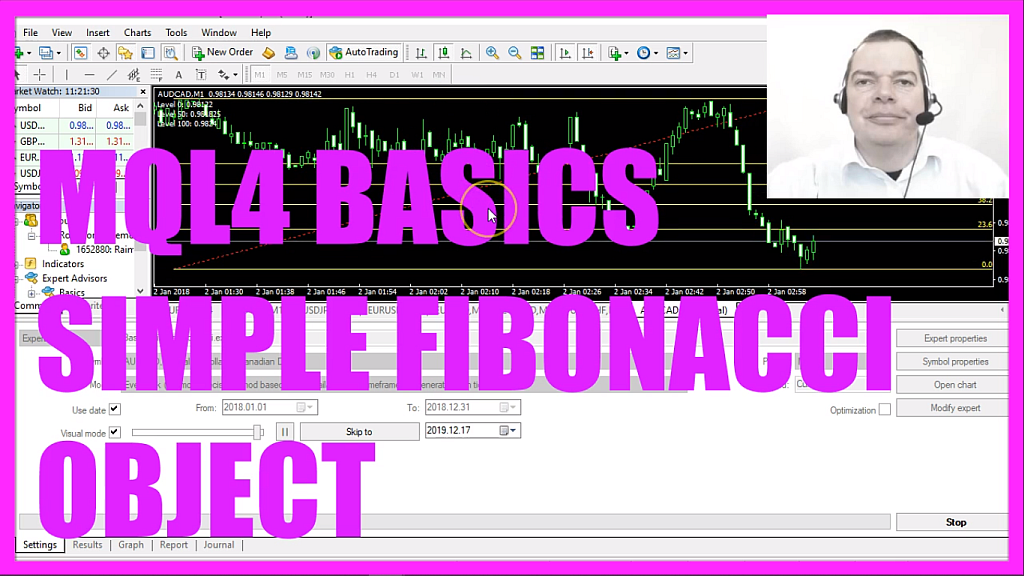In this video we are going to create a simple Fibonacci object on the chart, you see we have several Fibonacci levels here, so let’s find out how to do that with mql4.
To get started please click on a little button here or press F4 on your keyboard, now you should see the Metaeditor window and here you want to click on file, new file, expert advisor from template, continue, I will call this file simple Fibonacci object, click on continue, continue and finish.
Now you can delete everything above the on tick function and the two comment lines here.
This video was requested by one of the premium course members and to calculate the Fibonacci object for one hundred candles we first want to find the highest candle, this can be done by using the function ihighest if you mark that and press F1 you will see that it will return the maximum value over a specific number of bars. In our case we do that for the current symbol on the chart and the currently selected period on that chart, we use mode underscore high – all in capital letters – because mode underscore high will give us the high prices for each candle and we want to do that for one hundred candles starting with candle zero.
For the lower border of our Fibonacci object, we also want to know the lowest candle, that can be calculated by using ilowest, that’s also for the current symbol on the chart and the currently selected period, but this time we use mode underscore low to get the low prices for one hundred candles starting with candle zero.
In the next step, we use object delete to remove an object that is called Fibonacci, when we run the expert advisor for the very first time we don’t have such an object but afterwards we need to use object delete to remove the old object before we draw a new one and to do that we use object create, this function will create an object with a specified name type and some coordinates.
The first parameter here is the name of the object, I will call it Fibonacci, this is a string value, the second parameter is the type of the object, we are going to use obj underscore fibo, if you mark that and press F1 you will see that there are lots of other different object types.
The next value here is for the main chart, chart zero is the chart with the candles and we are going to draw right into the chart. For oscillators, you could also use a separate window below the candles.
The right border will be the current candle, for the upper border we want to use the highest price from the highest candle, we will use candle one hundred for the left border and the lowest price of the lowest of the one hundred candles will be the lower border.
Let’s actually calculate a few of those Fibonacci levels.
Level one hundred can be calculated by using object get double for the current main chart, we want to get the price for the Fibonacci object, the property is obj prop underscore price – all in capital letters –, let’s look that one up, there are a lot of different properties.
Let’s see if we can find some details, here we are! We have price one, price two, price three and so on.
We want to get the first price for this object to calculate level one hundred. To calculate level zero we do the same but this time the last parameter is one and level fifty will be calculated by adding the values of level zero and level one hundred and dividing the result by two, so let’s use a comment statement that will output the text followed by the calculated level for each of those three values.
Well if this was too fast for you or if you don’t understand what all the code here does maybe you want to watch one of the other videos in this basic video series or maybe even the premium course on our website might be interesting for you, for now, please click on the compile button here or press F7 on your keyboard, that should work without any errors and if that is the case you can click on a little symbol here or press F4 to go back to Metatrader.
And in Metatrader, we click on view, strategy tester or press control and r, please pick the new file simple Fibonacci object dot ex4, enable the visual mode here and start a test.
Here is our object, we can see the Fibonacci levels.
Let’s fast forward a little bit, stop the test, click the middle mouse button and see if the levels match. We have a value of eighty-eight dot zero three four, that’s also what we have calculated for level hundred here.
Level zero is eighty-seven dot nine one four, this is also true, so our little expert advisor is working and now you know how to calculate and code a Fibonacci object for Metatrader 4 and you have coded it yourself with a few lines of mql4 code.